Question

# You wish to make a 0.445 M hydrochloric acid solution from a stock solution of 12.0...

You wish to make a 0.445 M hydrochloric acid solution from a stock solution of 12.0 M hydrochloric acid. How much concentrated acid must you add to obtain a total volume of 150 mL of the dilute solution?

To obtain a total volume of 150 mL of the dilute solution we have to take 5.5 ml of stock solution

The solution is as follows,

step 1]we need to find how much acid should be in the final solution and then find the volume of initial solution containing that amount of acid:

0.445 M solution means that 1 L of solution contains 0.445 moles of acid. Then 150mL (0.150 L) of such solution contains (0.150 L/1L) * 0.445 mol = 0.06675 moles.

step 2] we also need to find the amount of stock solution containing 0.06675 moles of acid,

1 L of 12M solution contains 12 moles of acid. Then 0.06675 moles is in (0.06675 moles/12 moles) * 1L = 0.0055 L = 5.5 mL.

#### Earn Coins

Coins can be redeemed for fabulous gifts.

Similar Homework Help Questions
• ### You wish to make a 0.249 M hydrobromic acid solution from a stock solution of 12.0...

You wish to make a 0.249 M hydrobromic acid solution from a stock solution of 12.0 M hydrobromic acid. How much concentrated acid must you add to obtain a total volume of 50.0 mL of the dilute solution? __mL You wish to make a 0.112 M nitric acid solution from a stock solution of 12.0 M nitric acid. How much concentrated acid must you add to obtain a total volume of 150 mL of the dilute solution? __mL In the...

• ### You wish to make a 0.252 M nitric acid solution from a stock solution of 12.0...

You wish to make a 0.252 M nitric acid solution from a stock solution of 12.0 M nitric acid. How much-concentrated acid must you add to obtain a total volume of 175 mL of the dilute solution?

• ### 1b. You wish to make a 0.395 M perchloric acid solution from a stock solution of...

1b. You wish to make a 0.395 M perchloric acid solution from a stock solution of 3.00 M perchloric acid. How much concentrated acid must you add to obtain a total volume of 75.0 mL of the dilute solution? 1c. In the laboratory you dilute 4.36 mL of a concentrated 12.0 M perchloric acid solution to a total volume of 50.0 mL. What is the concentration of the dilute solution?

• ### You wish to make a 0.135 M nitric acid solution from a stock solution of 3.00...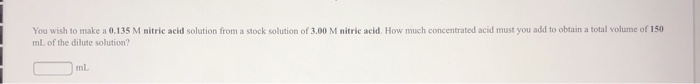You wish to make a 0.135 M nitric acid solution from a stock solution of 3.00 M nitric acid. How much concentrated acid must you add to obtain a total volume of 150 mL of the dilute solution? ml

• ### You wish to make a 0.257 M nitric acid solution from a stock solution of 3.00...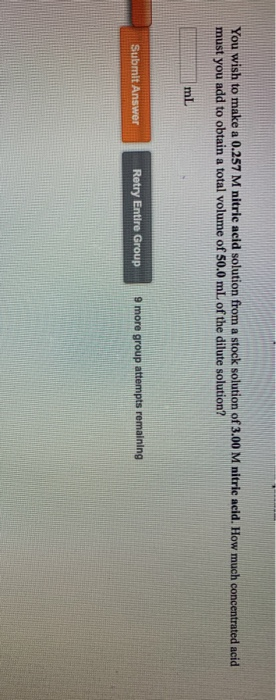You wish to make a 0.257 M nitric acid solution from a stock solution of 3.00 M nitric acid. How much concentrated acid must you add to obtain a total volume of 50.0 mL of the dilute solution? Submit Answer Retry Entire Group 9 more group attempts remaining

• ### You wish to make a 0.132 M nitric acid solution from a stock solution of 6.00...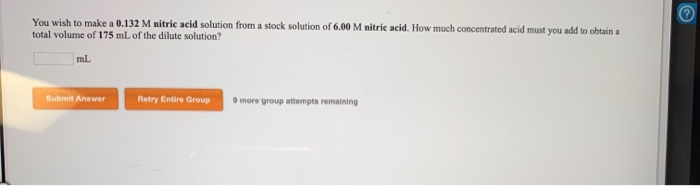You wish to make a 0.132 M nitric acid solution from a stock solution of 6.00 M nitric acid. How much concentrated acid must you add to obtain total volume of 175 mL of the dilute solution? Submit Answer Retry Entire Group more group attempts remaining 1 pts are In the laboratory a student combines 37.6 ml of a 0.289 M potassium sulfide solution with 22.5 ml of a 0.629 M potassium carbonate solution What is the final concentration of...

• ### In the laboratory, you dilute 3.61 mL of a concentrated 12.0 M hydrochloric acid solution to...

In the laboratory, you dilute 3.61 mL of a concentrated 12.0 M hydrochloric acid solution to a total volume of 175 mL. What is the concentration of the dilute solution?

• ### Gonca the References to importatif eded for the You wish to make a 0.266 M perchloric...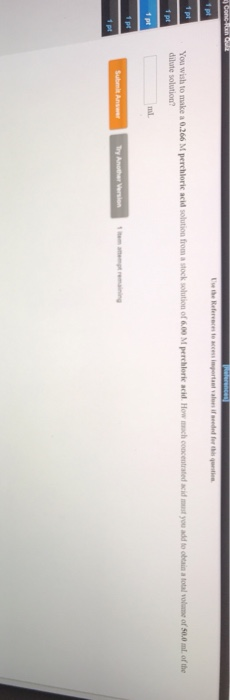Gonca the References to importatif eded for the You wish to make a 0.266 M perchloric acid solution from a stock solution of 6.00 M perchloric acid How much concentrated dilute solution you to obtain a lotal volume of 50.0 ml of the Try Another Version

• ### I do not need to see work shown, just the correct exact answers. Please be clear...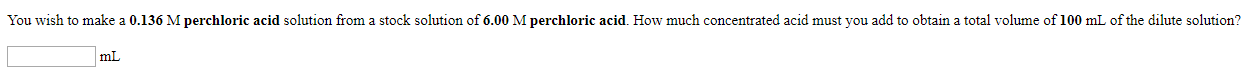I do not need to see work shown, just the correct exact answers. Please be clear on what the answers are. I will give thumbs up if correct. 4-10 You wish to make a 0.136 M perchloric acid solution from a stock solution of 6.00 M perchloric acid. How much concentrated acid must you add to obtain a total volume of 100 mL of the dilute solution? mt In the laboratory you dilute 4.47 mL of a concentrated 3.00 M...

• ### Name: ume of concentrated 12.0 M hydrochloric acid is needed to prepare 115.0 mL of a 3.25 M solution? Name: u...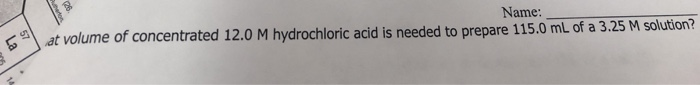Name: ume of concentrated 12.0 M hydrochloric acid is needed to prepare 115.0 mL of a 3.25 M solution? Name: ume of concentrated 12.0 M hydrochloric acid is needed to prepare 115.0 mL of a 3.25 M solution?